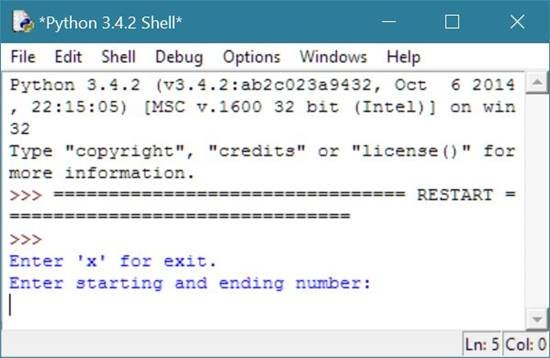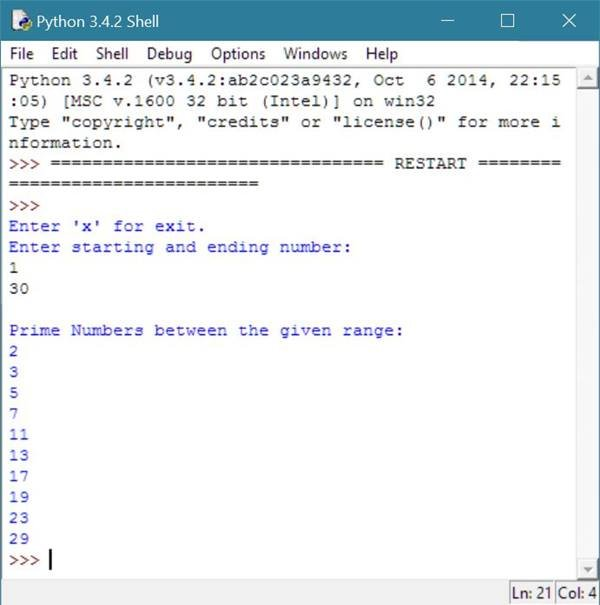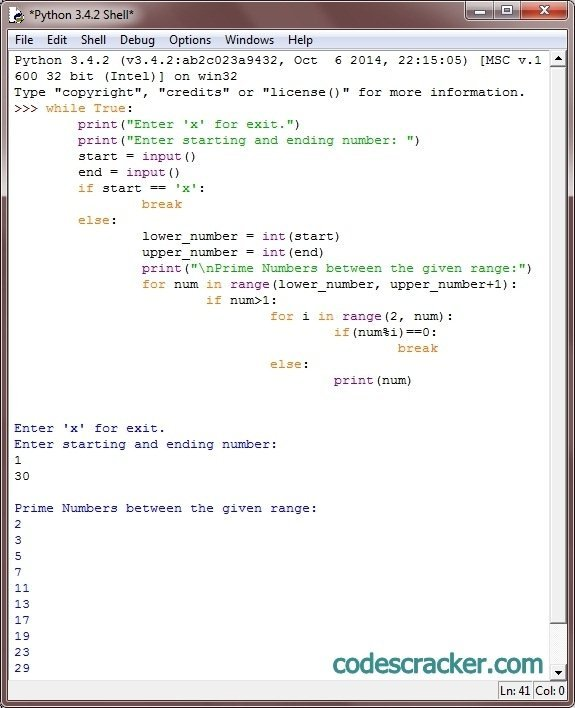# Python Program to Print Prime Numbers

## Print Prime Numbers in Python

To print prime numbers in python, you have to ask from user to enter the limit in which he/she want to print all the prime numbers. As you know that prime numbers are those numbers which are divisible by only 1 and the number itself.

Therefore, to print all the prime numbers between the given range, you have to check all the number in the given range, whether the number is prime or not, if the number is find to be prime number, then print that number and check for the next number until the range reached as shown in the program given below.

## Python Programming Code to Print Prime Numbers

Following python program ask from user to enter the range to print all the prime numbers present in the given range:

```# Python Program - Print Prime Numbers

print("Enter 'x' for exit.");
print("Enter starting and ending number: ");
start = input();
if start == 'x':
exit();
else:
end = input();
lower_number = int(start);
upper_number = int(end);
print("\nPrime Numbers between the given range:")
for num in range(lower_number, upper_number+1):
if num>1:
for i in range(2, num):
if(num%i)==0:
break;
else:
print(num);
```

Here is the sample run of the above python program to illustrates how to print all the prime numbers between the range provided by the user:Above sample initial output will ask from user to enter the two numbers to find all the prime numbers between the given two numbers. You must have to enter the first number which will be less than the second number, say 1 as first number and 30 as second number and then press enter as shown in the below screenshot:Here is the same program on python shell:### Same Program in Other Languages

You may also like to learn or practice the same program in other popular programming languages:

Tools
Calculator